# Model 2: A Chikungunya Model

A majority of my work so far has been in respitatory viruses and HIV, so developing models for vector-borne viruses is a fun challenge for me. Here, we have my take on a Chikungunya (CHIKV) model developed by Yakob & Clements for the 2006 Réunion Island outbreak.

I try a couple of different versions of the model to try to replicate the results here. This post is a short example of the process that goes into model development.

Yakob & Clements mention that there are approximately 785,000 inhabitants on Réunion Island so I assume that this is the population size they use.

The authors do not include the mosquito population size used in their model. Brazil has 10-250 million mosquitos according to Suprarit et al., so I use 9 million for Reunion Island initially.

I disregard the omega variable in this analysis because quantifying the number of symptomatic individuals is not important to us here.

References:

Yakob L, Clements AC. A mathematical model of chikungunya dynamics and control: the major epidemic on Réunion Island. PLoS One. 2013;8(3):e57448. doi:10.1371/journal.pone.0057448

Suparit P, Wiratsudakul A, Modchang C. A mathematical model for Zika virus transmission dynamics with a time-dependent mosquito biting rate. Theor Biol Med Model. 2018;15(1):11. Published 2018 Aug 1. doi:10.1186/s12976-018-0083-z

## Model A

``````chikv <-function(t, t0, parms) {
with(as.list(c(t0, parms)), {

num <- s.num + e.num + i.num + ia.num + r.num
num.m <- x.num + y.num + z.num

dS <- -1*beta1*(s.num)*(z.num / num.m)
dE <- (beta1*(s.num)*(z.num / num.m)) - (lambda1*(e.num))
dI <- (theta*lambda1*(e.num)) - (gamma*(i.num))
dIa <- ((1 - theta)*lambda1*(e.num)) - (gamma*(ia.num))
dR <- gamma*((i.num + ia.num))

dX <- (mu*x.num) - (beta2*(x.num)*((i.num+ia.num) / num)) - (mu*(x.num))
dY <- (beta2*(x.num)*((i.num+ia.num) / num)) - (lambda2*(y.num)) - (mu*(y.num))
dZ <- (lambda2*(y.num)) - (mu*(z.num))

list(c(dS,
dE,
dI,
dIa,
dR,
dX,
dY,
dZ,
se.flow <- (beta1*s.num*(z.num / num.m)),
ei.flow <- (lambda1*e.num),
ir.flow<- gamma*(i.num + ia.num),
xy.flow <- (beta2*x.num*((i.num + ia.num) / num)),
yz.flow <- (lambda2*y.num)
))
})
}``````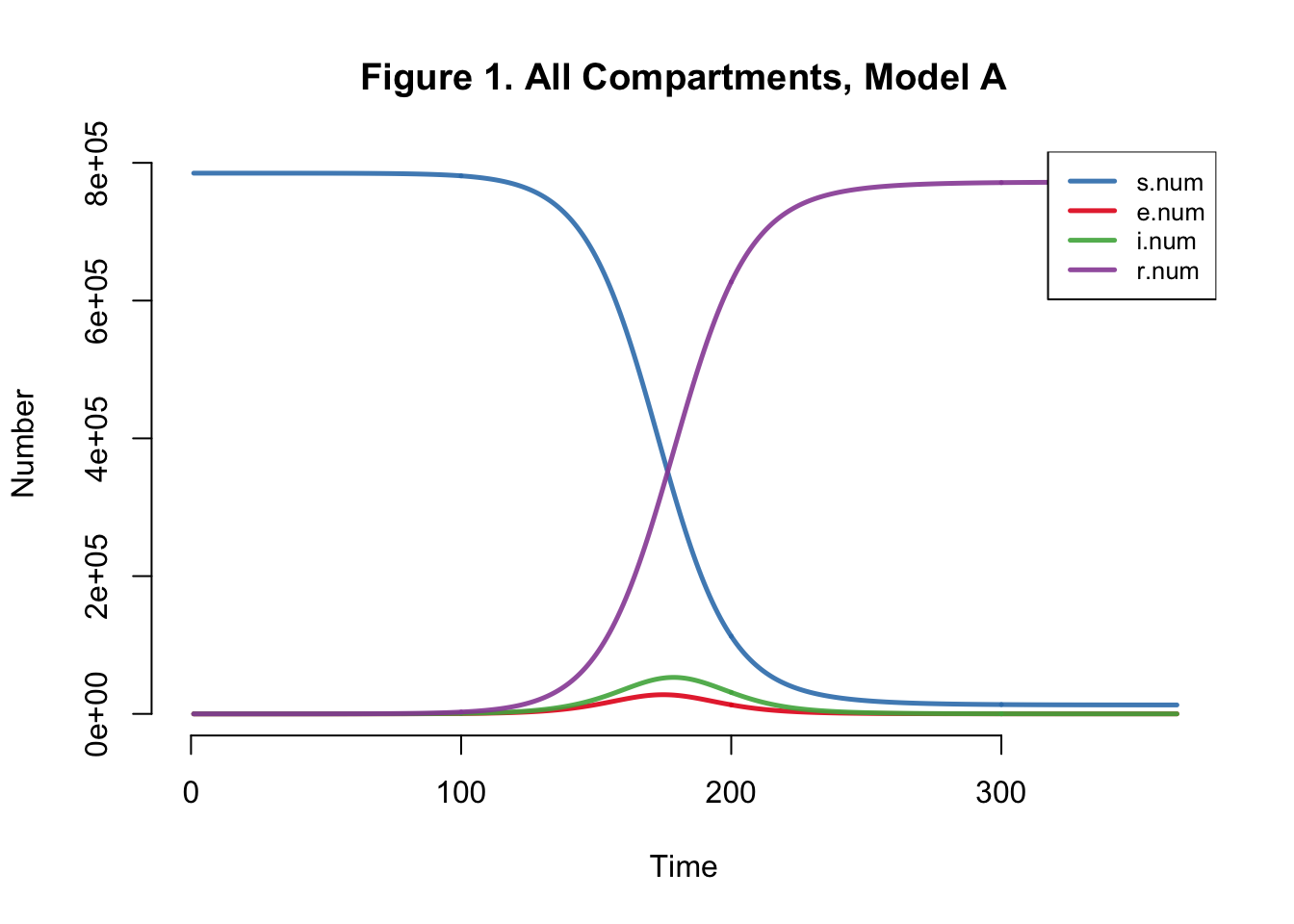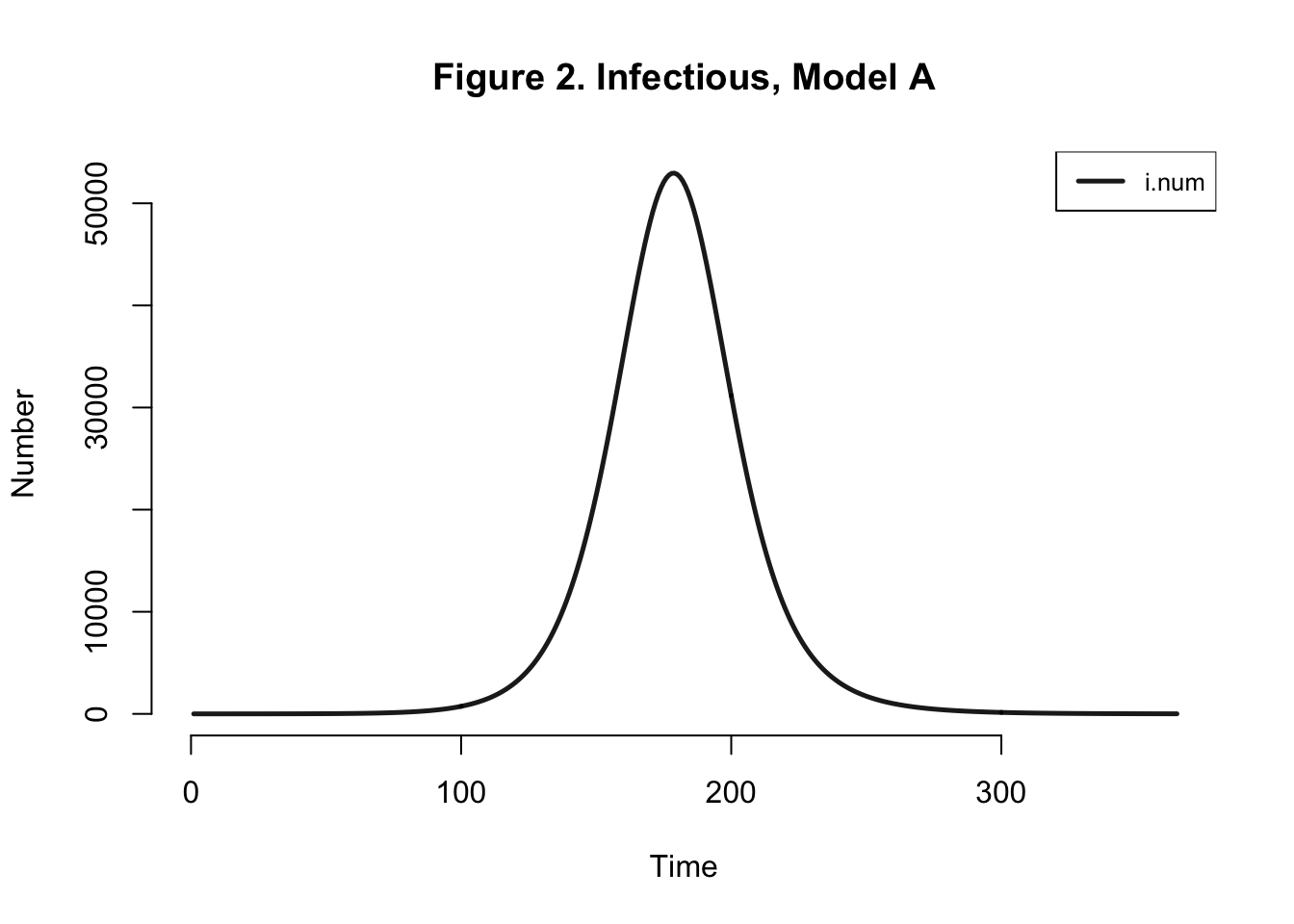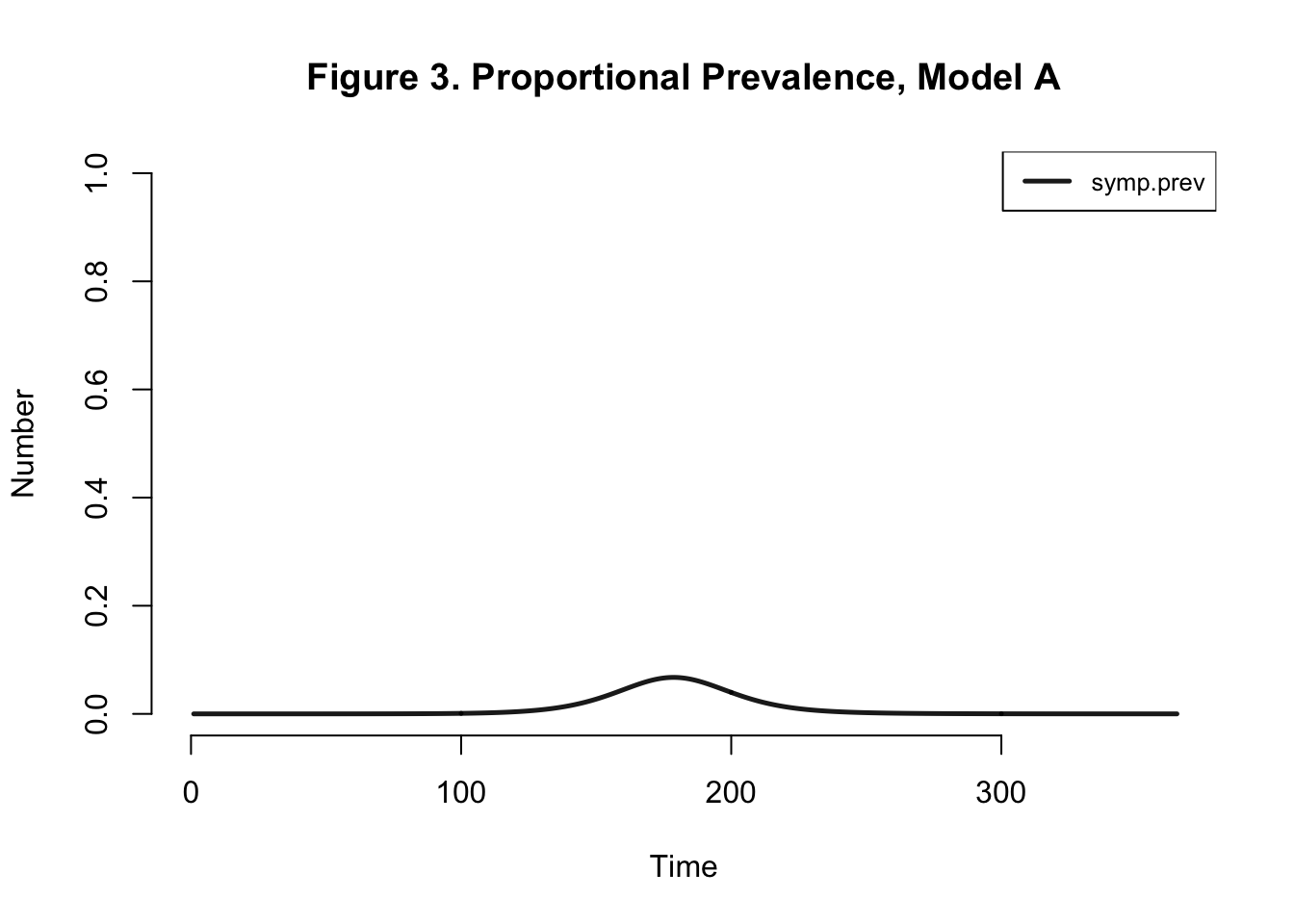Table 1: Model A Outcomes
Peak.Incidence Peak.Prevalence Total.Infected
1.8% 6.7% 98.4%

Peak Incidence here is not consistent with the paper’s findings (5.3%). Final attack rate should be around 42%, not 98.4% as we found here. I’ve only done a deterministic analysis, I still feel that I should be getting an attack rate similar to that of the authors.

Upon taking another look at the model, it doesn’t really make sense to me that only uninfected mosquitos (in the X compartment) would be breeding. Wouldn’t mosquitos in all compartments procreate?

I change this below:

## Model B

``````chikv <-function(t, t0, parms) {
with(as.list(c(t0, parms)), {

num <- s.num + e.num + i.num + ia.num + r.num
num.m <- x.num + y.num + z.num

dS <- -1*beta1*(s.num)*(z.num / num.m)
dE <- (beta1*(s.num)*(z.num / num.m)) - (lambda1*(e.num))
dI <- (theta*lambda1*(e.num)) - (gamma*(i.num))
dIa <- ((1 - theta)*lambda1*(e.num)) - (gamma*(ia.num))
dR <- gamma*((i.num + ia.num))

dX <- (mu*num.m) - (beta2*(x.num)*((i.num+ia.num) / num)) - (mu*(x.num))
dY <- (beta2*(x.num)*((i.num+ia.num) / num)) - (lambda2*(y.num)) - (mu*(y.num))
dZ <- (lambda2*(y.num)) - (mu*(z.num))

list(c(dS,
dE,
dI,
dIa,
dR,
dX,
dY,
dZ,
se.flow <- (beta1*s.num*(z.num / num.m)),
ei.flow <- (lambda1*e.num),
ir.flow<- gamma*(i.num + ia.num),
xy.flow <- (beta2*x.num*((i.num + ia.num) / num)),
yz.flow <- (lambda2*y.num)
))
})
}``````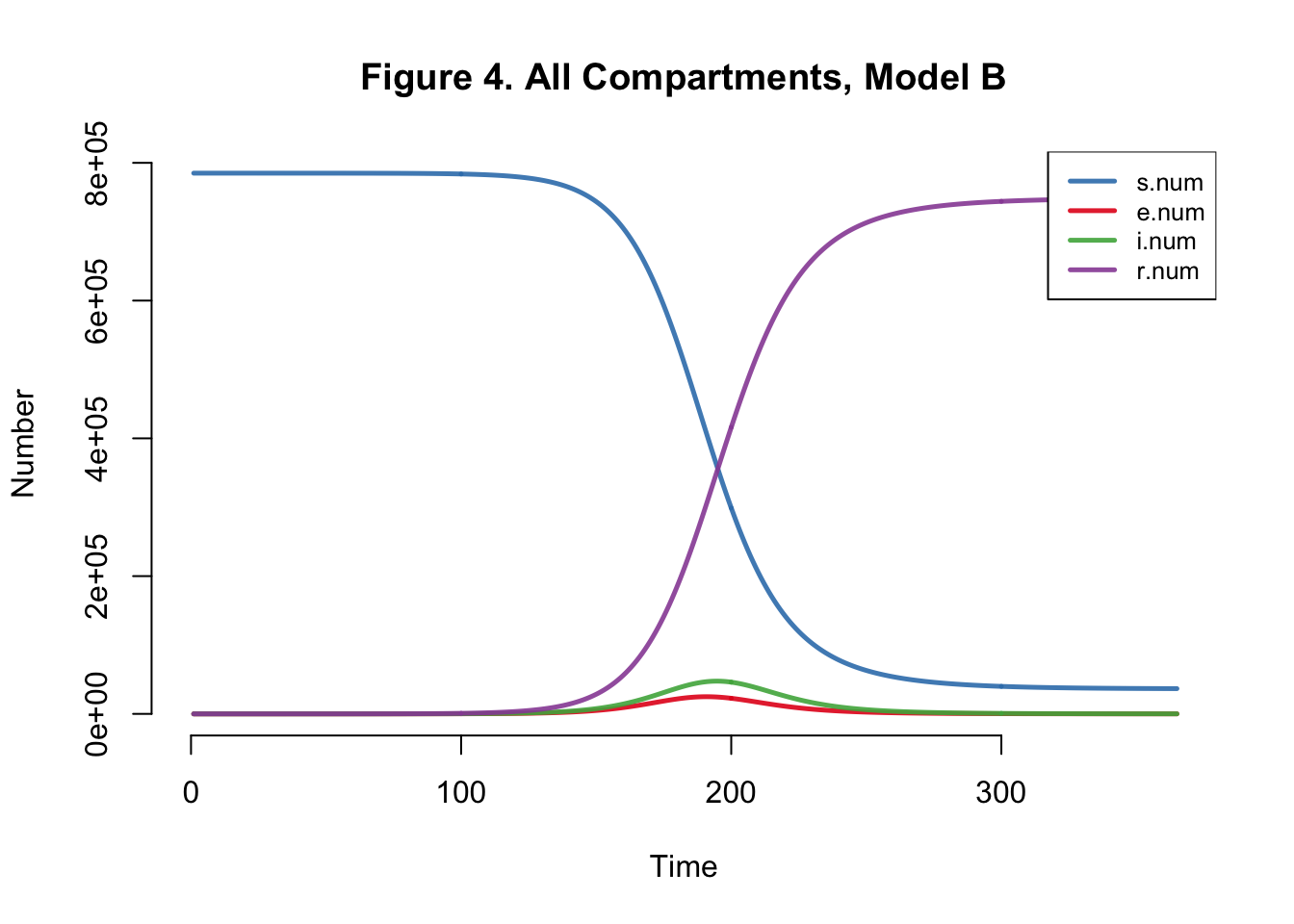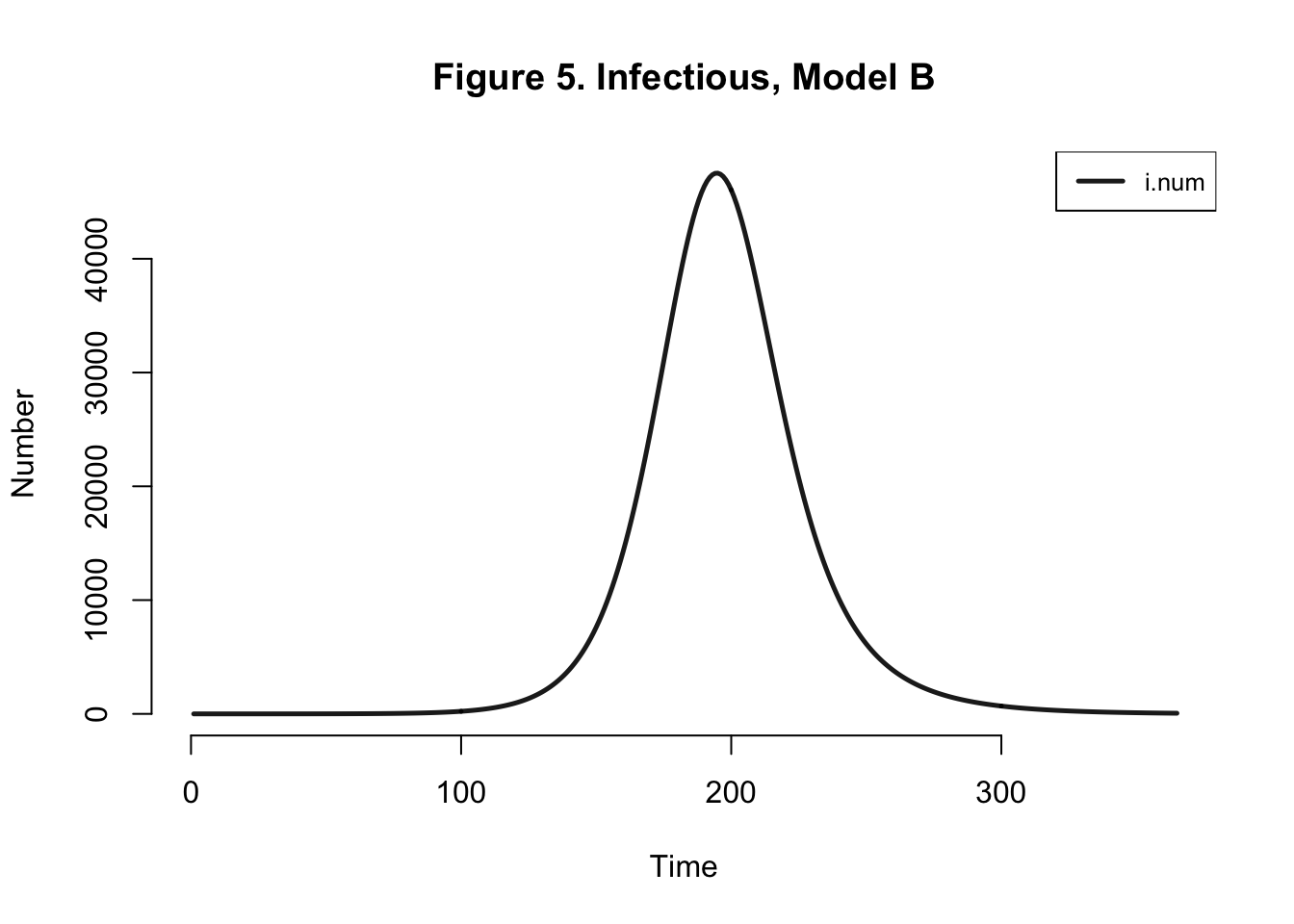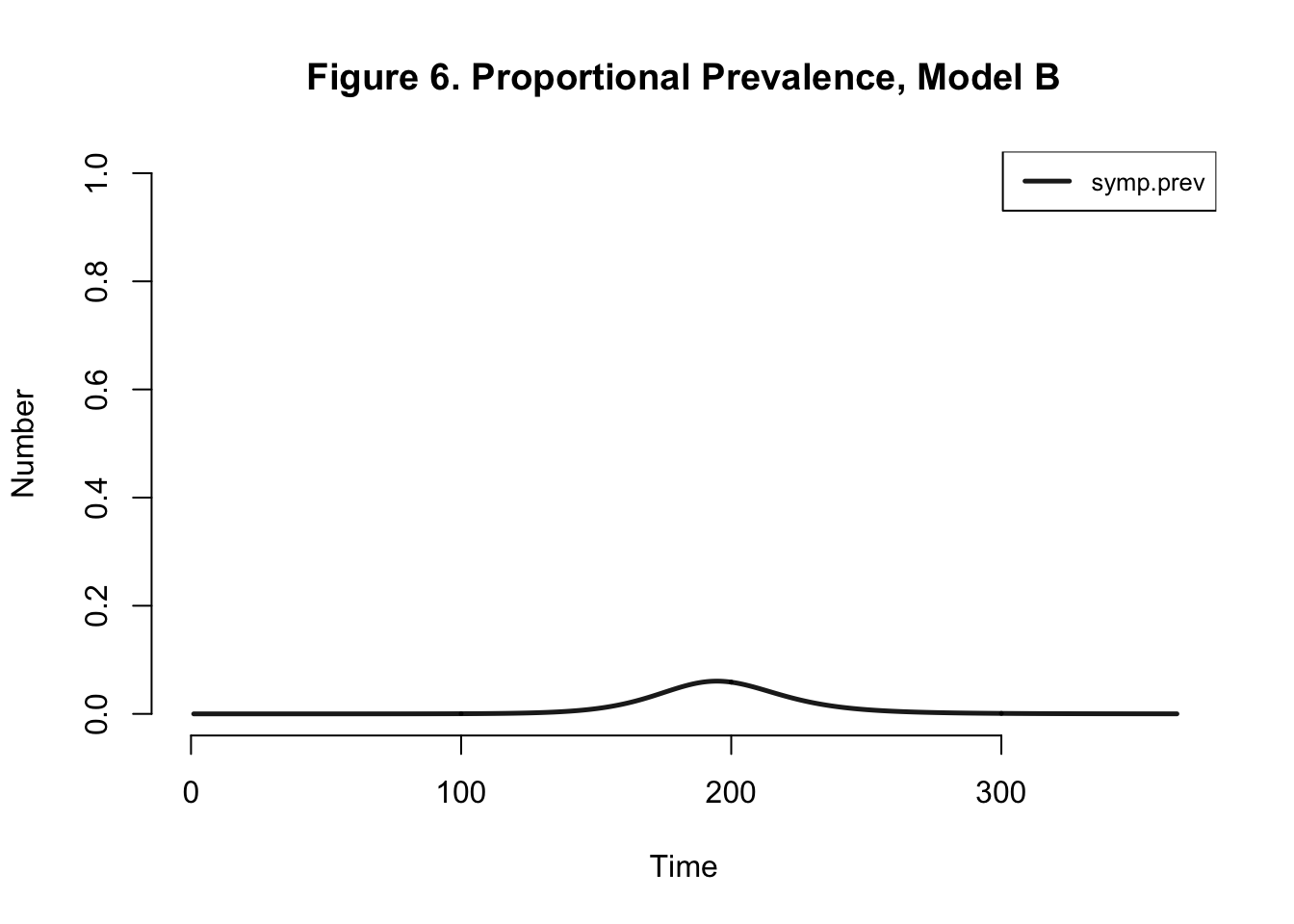Table 2: Model B Outcomes
Peak.Incidence Peak.Prevalence Total.Infected
1.6% 6.1% 95.3%

Peak prevalence and peak incidence are actually slightly reduced here - because we introduce more uninfected susceptibles than in the prior model if I assume that all mosquitos breed to create suseceptible mosquitos.

Either way, our EpiModel version of this chikungunya model is grossly overestimating the transmission process… Let’s try altering the mosquito population - from 9 million to 785,000. One mosquito per human.

## Model C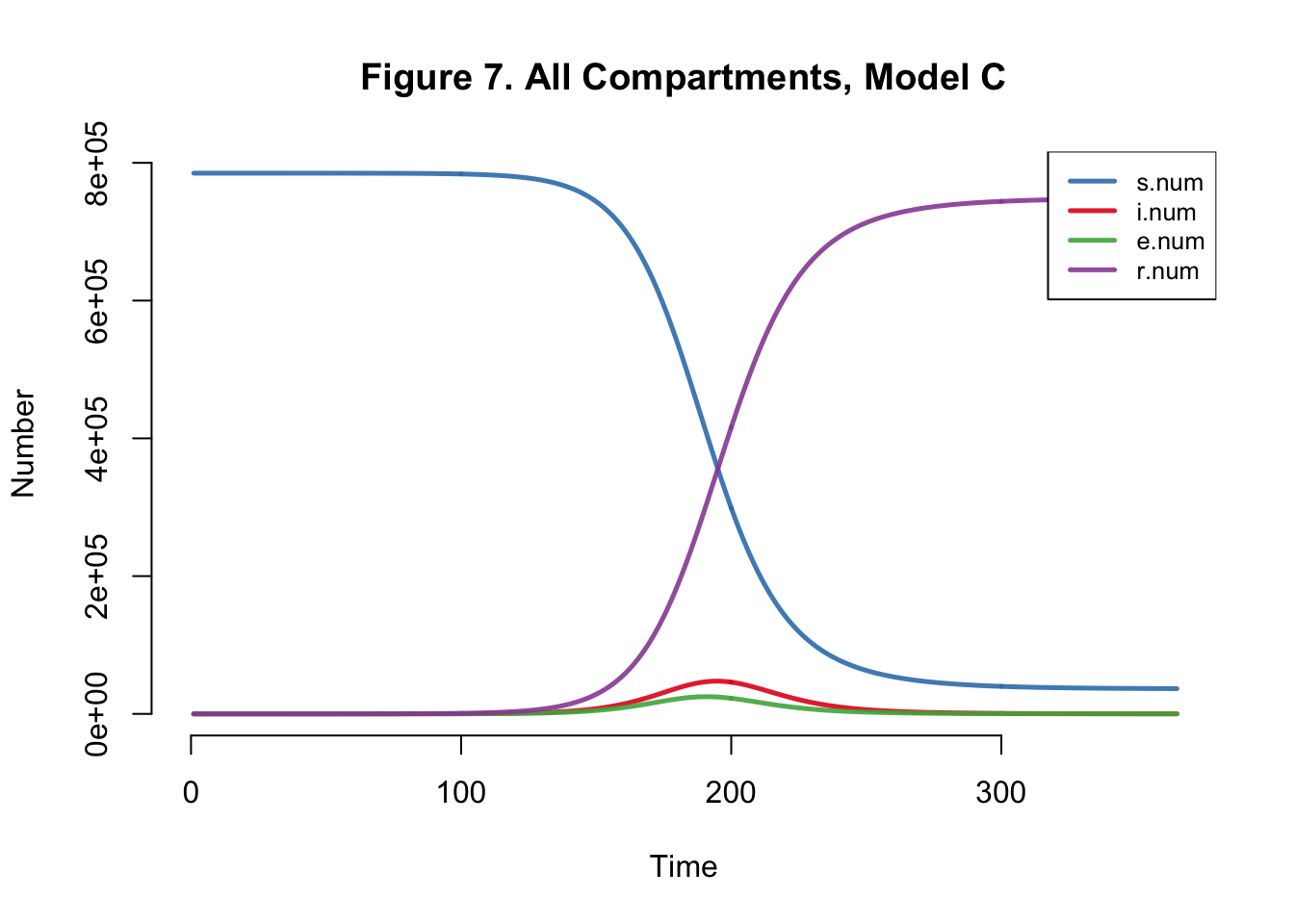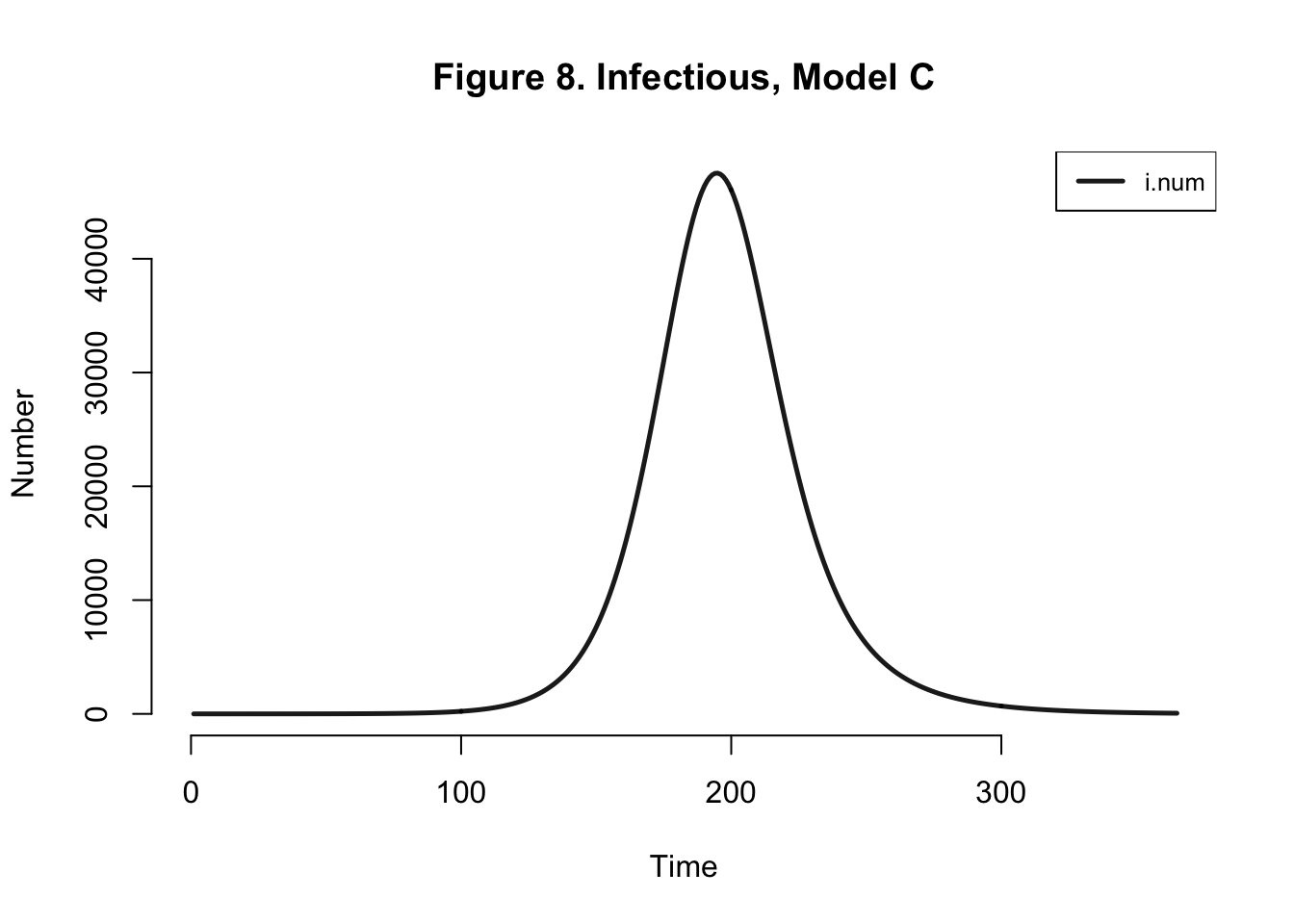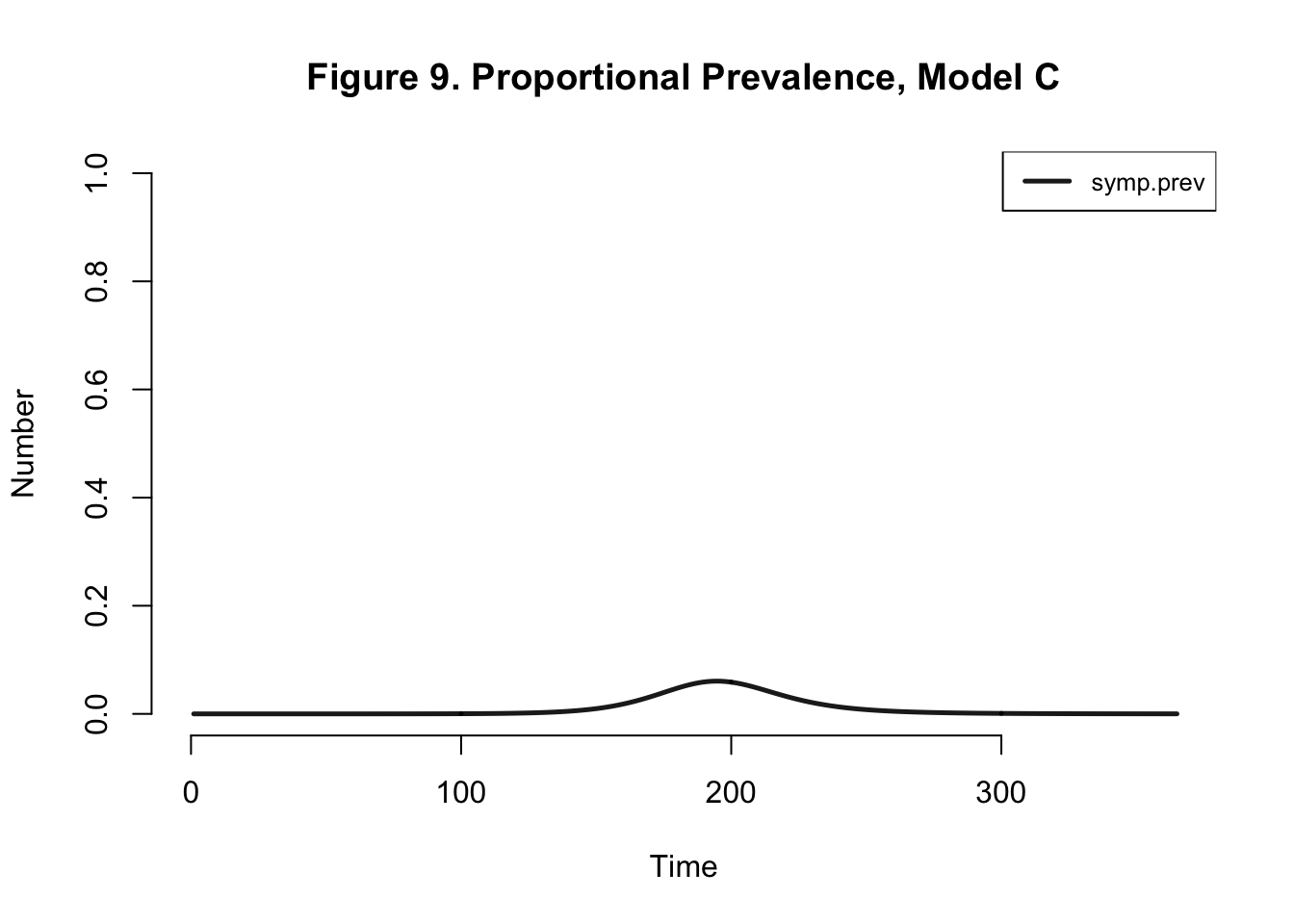Table 3: Model C Outcomes
Peak.Incidence Peak.Prevalence Total.Infected
1.6% 6.1% 95.3%

Even if I edit the number of mosquitos in the population but keep the model the same, we get the same dynamics within the human population.

This is an example of how infectious disease models are not always replicable because of interpretation of parameters and underlying methods. It is actually important to have models that acknowledge different nuances that are left out in others to show a range of outcomes.

But, this is why using multiple models is a MUST if we’re relying on models to make important policy or intervention decisions. And a plus would be publicly available code for those models!

I’ll eventually recreate some COVID-19 models that highlight the same message.